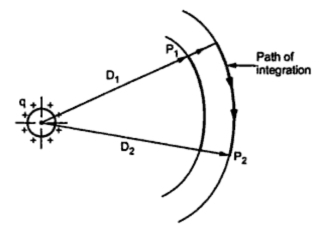### Potential Difference between Two Points due to a Charge

The potential difference between any two points is nothing but the work done in joules per coulomb required to move a coulomb of charge between the two points. The force on a charge in the field is measured by electric field intensity which is equal to the force in newtons per coulomb on a coulomb charge at the point considered and is measured in volts per meter.
Consider a long straight wire carrying positive charge of q c/m as shown in the Fig. 1.Fig. 1
Consider two points P1 and P2 located at a distance of  D1 and D2 from centre of wire. There is positive charge on wire which will repel when a positive charge is placed in the field. If we want to move charge from point P2 to P1 then work must be done on positive charge. Here P1 is at higher potential than P2. If the charge moves from P1 to P2, it expends energy which is nothing but voltage drop from P1 to P2. The path followed does not affect the potential difference.
In order to find the voltage drop from P1 to P2 is to obtain the voltage between equipotential surfaces passing through P1 and P2.
The voltage drop between P1 and P2 is,
The voltage drop between two points may be either positive or negative depending upon the charge causing the potential difference is positive or negative. It also depends upon whether the voltage drop is computed from a point near the conductor to a point far away or vice versa.